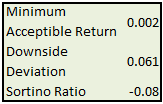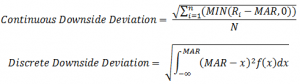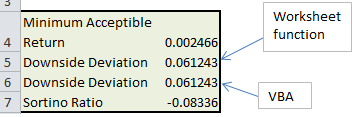# Downside Deviation as a Risk Measure

This article provides an Excel spreadsheet to calculate downside deviation (including VBA and a matrix formula). It also discusses why downside deviation is a better risk measure than the standard deviation.

## Standard Deviation as a Proxy for Risk

Standard deviation is commonly used as a measure of investment risk, and is typically employed when calculating performance benchmarks like the Sharpe Ratio. Standard deviation describes the variability around the mean of an investment’s returns, with higher values indicating an investment whose returns have a large spread and hence a greater risk.But standard deviation has several shortcomings as a proxy for risk. Standard deviation

• includes both variation above the mean below the mean.  This means that returns that spike heavily above the mean are considered bad.This behavior does not, however, model the risk preferences of most investors. Most investors only consider variation below an acceptable return as “bad” risk
• assumes that the returns are symmetric. This is not valid for exotic investments techniques like options or short selling.
• assumes that all investors, from retirees to hedge fund managers, have the same definition of risk

## Downside Deviation as a Superior Risk Measure

Sortino proposed the downside deviation as an alternative that better models the risk preferences of most investors. Downside deviation is a modification of the standard deviation such that only variation below a minimum acceptable return is considered. The minimum acceptable return can be chosen to match specific investment objectives.

Downside deviation for discrete and continuous returns is defined by the following equationsRi are the investment returns, MAR is the minimum acceptable return, and f(x) is the continuous probability distribution of the returns.

We now present two methods of calculating the downside deviation in Excel; the first uses an Excel matrix formula, and the second uses VBA. Both approaches give the same results.## Calculate Downside Deviation in Excel

Calculating the downside deviation for discrete returns data using an Excel matrix formula is simple. In the following formula, cells C10:C21 represent the returns and cell B4 represents the minimum acceptable return.

`{=STDEV.P(IF(C10:C21<B4,C10:C21,""))}`

The curly braces {} indicate a matrix formula entered with CTRL+SHIFT+ENTER.

## Calculate Downside Deviation in VBA

The first argument is a range of returns, and the second argument is the minimum acceptable return.

```Function DownsideDev(returns As Range, MAR As Variant) As Double
Dim cell As Range
Dim avg As Double, temp1 As Double, temp2 As Double
i = 0
temp1 = 0
temp2 = 0
For Each cell In returns
If cell.Value < MAR Then
temp1 = temp1 + cell.Value
i = i + 1
End If
Next cell
avg = temp1 / i
temp = 0
For Each cell In returns
If cell.Value < MAR Then
temp2 = temp2 + (cell.Value - avg) ^ 2
End If
Next cell
DownsideDev = Sqr(temp2 / i)
End Function```

This spreadsheet implements both methods. It also calculates the Sortino Ratio, a modification of the Sharpe Ratio that used downside deviation as a proxy for risk.

Download Excel Spreadsheet to Calculate Downside Deviation

## 10 thoughts on “Downside Deviation as a Risk Measure”

1. Pingback: Downside Deviation
2. ASHES says:

=STDEV.P(IF(C10:C21<B4,C10:C21,"")) is wrong.
it will test C10, if C10<B4, it will calculate standard deviation from C10 to C21, otherwise, u get an error cuz "" doesn't have standard deviation.

1. ASHES says:

not the first value, but the box which is at the same row with the box u put ur formula. if no value in the same row, u get a not valid number!

2. Bear in mind that the formula is {=STDEV.P(IF(C10:C21

3. Artur says:

Hi, Thanks for this very useful post. However, I think that the downside deviation is not calculated correctly. To cut the long story short, when you use the array formula in Excel, the value for N will be the number of values that meet your acceptable minimum return, rather than all values, which is the correct way to do it as far as I know. For instance, if you have 5 returns and 3 meet you requirements, then the array formula will use 3 in the denominator, rather than 5 (or 5-1, for sample data).

1. I’ve seen both methods used to calculate the downside deviation. There is no strictly correct mathematical defined method. Just use a consistent method..

4. Artur says:

What about the fact that the formula that you provide subtracts average from every data point that qualifies for your sample. This means that you are not calculating semi-standard deviation below the target (B4) in your example.

Here is an example:

Dataset: 1; -3; -5; 2; 5; 8; -1
Target (MAR): 0

Excel standard deviation of points -3, – 5 and -1 returns 2. This is what your array formula does. Now, to arrive at 2, Excel subtracts the AVERAGE from each of the 3 data points. This means that you are not calculating the semi-standard deviation for the target of 0, but for the target of -3.

The correct answer as to what is the semi-standard deviation below the target of 0 is not 2, as with your formula, but 4.1833. The fact that the semi-standard deviation below the target of 0 is more than 2 is easily visible from the dataset alone.

1. Sal says:

Artur-
you are correct. If you want to calculate downside deviation in a single cell as an array, the formula should be:

{=SQRT(SUM(IF(A1:A20<\$B\$1,((A1:A20)-\$B\$1)^2,0))/COUNT(A1:A20))}
where your Min Acceptable Return (MAR) is in B1

This subtracts the correct MAR from each observation and divides by the count of all observations.

5. Alex says:

hey guys!
can i also use a range of the minimum acceptable reuturns instead of just one generic figure?
let’s say i have daily data on a risk-free asset which i want to use as my minimum accpetable reuturn.
in other words:
could i just simply make this:
=STDEV.P(IF(C10:C21<B4,C10:C21,""))
into this
=STDEV.P(IF(C10:C21<B10:B21,C10:C21,""))
assuming column C is the range of returns of my portfolio
& column B is the range of returns of my minimum acceptable returns?

please help!!! (writing my dissertation right now on this…)

6. ORCoder says:

1) I am pretty sure that the label for continuous and discrete downside risk are backwards- you can take sums of discrete things and integrals of continuous things, but the item marked “Continuous Downside Deviation” has a sum while the item marked “Discrete Downside Deviation” has an integral.

2) The formula with the summation (marked “Continuous Downside Deviation” , even though it is probably supposed to be marked “Discrete”) should have the MIN(R-MAR,0) term squared, (so it should have (R-MAR)^2).
Otherwise it doesn’t make sense to take the square root. Either the measure is supposed to be looking at absolute average downside deviation, and therefore should not muck around with with squares and squareroots at all, or it is supposed to be an downside analogue of standard deviation, in which case each term should be squared and the summation of the squared terms should be square rooted. Given that there are square and square roots in the other formula I am thinking it is the latter.